Spectrum¶

Colour is defined as the characteristic of visual perception that can be described by attributes of hue, brightness (or lightness) and colourfulness (or saturation or chroma).

When necessary, to avoid confusion between other meanings of the word, the term "perceived colour" may be used.

Perceived colour depends on the spectral distribution of the colour stimulus, on the size, shape, structure and surround of the stimulus area, on the state of adaptation of the observer's visual system, and on the observer's experience of the prevailing and similar situations of observation. 

Light is the electromagnetic radiation that is considered from the point of view of its ability to excite the human visual system. 

The portion of the electromatic radiation frequencies perceived in the approximate wavelength range 360-780 nanometres (nm) is called the visible spectrum.

In :
import colour

from colour.plotting import *
In :
colour_style();
In :
# Plotting the visible spectrum.
plot_visible_spectrum();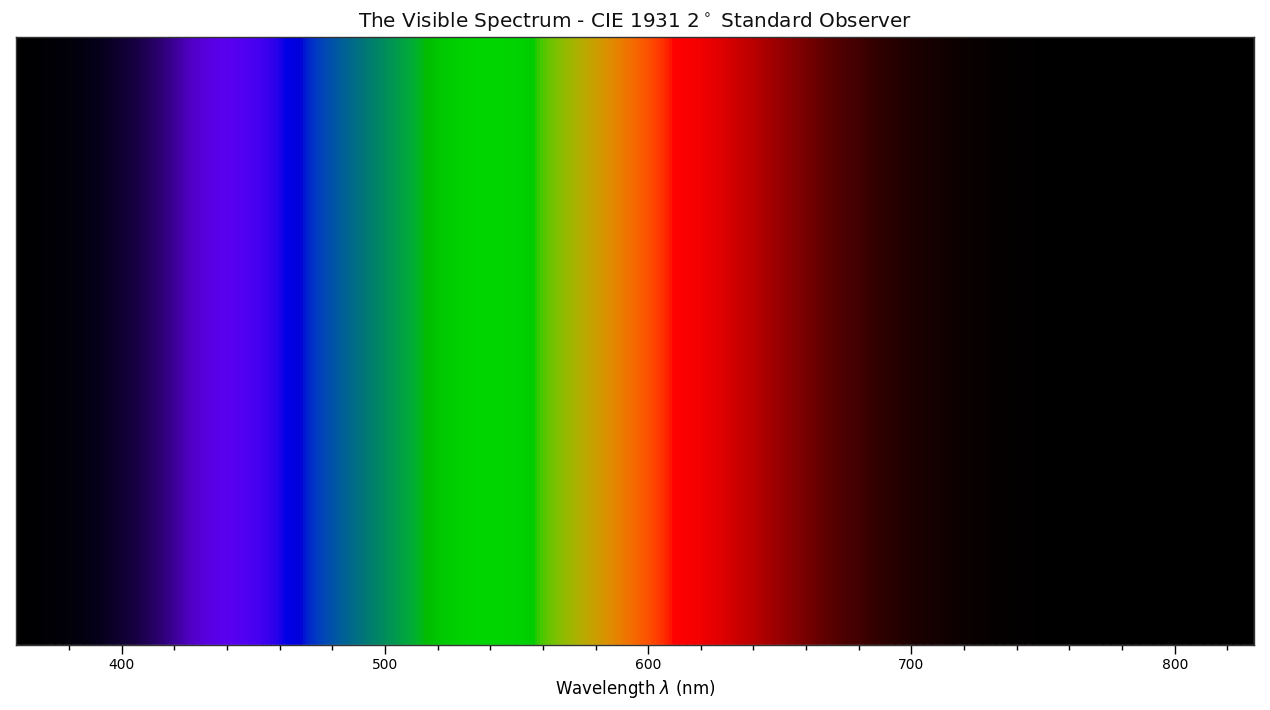The spectrum is defined as the display or specification of the monochromatic components of the radiation considered. 

At the core of Colour is the colour.colorimetry sub-package, it defines the objects needed for spectral related computations and many others:

In :
from pprint import pprint

import colour.colorimetry as colorimetry

pprint(colorimetry.__all__)
['SpectralShape',
'DEFAULT_SPECTRAL_SHAPE',
'SpectralDistribution',
'MultiSpectralDistributions',
'sds_and_multi_sds_to_sds',
'sd_blackbody',
'planck_law',
'LMS_ConeFundamentals',
'RGB_ColourMatchingFunctions',
'XYZ_ColourMatchingFunctions',
'CMFS',
'LMS_CMFS',
'RGB_CMFS',
'STANDARD_OBSERVERS_CMFS',
'ILLUMINANTS',
'D_ILLUMINANTS_S_SDS',
'HUNTERLAB_ILLUMINANTS',
'ILLUMINANTS_SDS',
'LIGHT_SOURCES',
'LIGHT_SOURCES_SDS',
'LEFS',
'PHOTOPIC_LEFS',
'SCOTOPIC_LEFS',
'sd_constant',
'sd_zeros',
'sd_ones',
'SD_GAUSSIAN_METHODS',
'sd_gaussian',
'sd_gaussian_normal',
'sd_gaussian_fwhm',
'SD_SINGLE_LED_METHODS',
'sd_single_led',
'sd_single_led_Ohno2005',
'SD_MULTI_LEDS_METHODS',
'sd_multi_leds',
'sd_multi_leds_Ohno2005',
'SD_TO_XYZ_METHODS',
'MULTI_SD_TO_XYZ_METHODS',
'sd_to_XYZ',
'multi_sds_to_XYZ',
'ASTME308_PRACTISE_SHAPE',
'lagrange_coefficients_ASTME2022',
'tristimulus_weighting_factors_ASTME2022',
'sd_to_XYZ_integration',
'sd_to_XYZ_tristimulus_weighting_factors_ASTME308',
'sd_to_XYZ_ASTME308',
'multi_sds_to_XYZ_integration',
'multi_sds_to_XYZ_ASTME308',
'wavelength_to_XYZ',
'BANDPASS_CORRECTION_METHODS',
'bandpass_correction',
'bandpass_correction_Stearns1988',
'sd_CIE_standard_illuminant_A',
'sd_CIE_illuminant_D_series',
'daylight_locus_function',
'sd_mesopic_luminous_efficiency_function',
'mesopic_weighting_function',
'LIGHTNESS_METHODS',
'lightness',
'lightness_Glasser1958',
'lightness_Wyszecki1963',
'lightness_CIE1976',
'lightness_Fairchild2010',
'lightness_Fairchild2011',
'intermediate_lightness_function_CIE1976',
'LUMINANCE_METHODS',
'luminance',
'luminance_Newhall1943',
'luminance_ASTMD1535',
'luminance_CIE1976',
'luminance_Fairchild2010',
'luminance_Fairchild2011',
'intermediate_luminance_function_CIE1976',
'dominant_wavelength',
'complementary_wavelength',
'excitation_purity',
'colorimetric_purity',
'luminous_flux',
'luminous_efficiency',
'luminous_efficacy',
'RGB_10_degree_cmfs_to_LMS_10_degree_cmfs',
'RGB_2_degree_cmfs_to_XYZ_2_degree_cmfs',
'RGB_10_degree_cmfs_to_XYZ_10_degree_cmfs',
'LMS_2_degree_cmfs_to_XYZ_2_degree_cmfs',
'LMS_10_degree_cmfs_to_XYZ_10_degree_cmfs',
'WHITENESS_METHODS',
'whiteness',
'whiteness_Berger1959',
'whiteness_Taube1960',
'whiteness_Stensby1968',
'whiteness_ASTME313',
'whiteness_Ganz1979',
'whiteness_CIE2004',
'YELLOWNESS_METHODS',
'yellowness',
'yellowness_ASTMD1925',
'yellowness_ASTME313']

Note: colour.colorimetry sub-package public API is directly available from colour namespace.

Colour computations are based on a comprehensive dataset available in pretty much each sub-packages, for example colour.colorimetry.dataset defines the following data:

In :
import colour.colorimetry.datasets as datasets

pprint(datasets.__all__)
['CMFS',
'LMS_CMFS',
'RGB_CMFS',
'STANDARD_OBSERVERS_CMFS',
'ILLUMINANTS',
'D_ILLUMINANTS_S_SDS',
'HUNTERLAB_ILLUMINANTS',
'ILLUMINANTS_SDS',
'LIGHT_SOURCES',
'LIGHT_SOURCES_SDS',
'LEFS',
'PHOTOPIC_LEFS',
'SCOTOPIC_LEFS']

Note: colour.colorimetry.dataset sub-package public API is directly available from colour namespace.

Spectral Distribution¶

Whether it be a sample spectral distribution, colour matching functions or illuminants, spectral data is manipulated using an object built with the colour.SpectralDistribution class or based on it:

In :
import colour

# Defining a sample spectral distribution data.
sample_sd_data = {
380: 0.048,
385: 0.051,
390: 0.055,
395: 0.06,
400: 0.065,
405: 0.068,
410: 0.068,
415: 0.067,
420: 0.064,
425: 0.062,
430: 0.059,
435: 0.057,
440: 0.055,
445: 0.054,
450: 0.053,
455: 0.053,
460: 0.052,
465: 0.052,
470: 0.052,
475: 0.053,
480: 0.054,
485: 0.055,
490: 0.057,
495: 0.059,
500: 0.061,
505: 0.062,
510: 0.065,
515: 0.067,
520: 0.070,
525: 0.072,
530: 0.074,
535: 0.075,
540: 0.076,
545: 0.078,
550: 0.079,
555: 0.082,
560: 0.087,
565: 0.092,
570: 0.100,
575: 0.107,
580: 0.115,
585: 0.122,
590: 0.129,
595: 0.134,
600: 0.138,
605: 0.142,
610: 0.146,
615: 0.150,
620: 0.154,
625: 0.158,
630: 0.163,
635: 0.167,
640: 0.173,
645: 0.180,
650: 0.188,
655: 0.196,
660: 0.204,
665: 0.213,
670: 0.222,
675: 0.231,
680: 0.242,
685: 0.251,
690: 0.261,
695: 0.271,
700: 0.282,
705: 0.294,
710: 0.305,
715: 0.318,
720: 0.334,
725: 0.354,
730: 0.372,
735: 0.392,
740: 0.409,
745: 0.420,
750: 0.436,
755: 0.450,
760: 0.462,
765: 0.465,
770: 0.448,
775: 0.432,
780: 0.421}

sd = colour.SpectralDistribution(sample_sd_data, name='Sample')
print(sd)
[[  3.80000000e+02   4.80000000e-02]
[  3.85000000e+02   5.10000000e-02]
[  3.90000000e+02   5.50000000e-02]
[  3.95000000e+02   6.00000000e-02]
[  4.00000000e+02   6.50000000e-02]
[  4.05000000e+02   6.80000000e-02]
[  4.10000000e+02   6.80000000e-02]
[  4.15000000e+02   6.70000000e-02]
[  4.20000000e+02   6.40000000e-02]
[  4.25000000e+02   6.20000000e-02]
[  4.30000000e+02   5.90000000e-02]
[  4.35000000e+02   5.70000000e-02]
[  4.40000000e+02   5.50000000e-02]
[  4.45000000e+02   5.40000000e-02]
[  4.50000000e+02   5.30000000e-02]
[  4.55000000e+02   5.30000000e-02]
[  4.60000000e+02   5.20000000e-02]
[  4.65000000e+02   5.20000000e-02]
[  4.70000000e+02   5.20000000e-02]
[  4.75000000e+02   5.30000000e-02]
[  4.80000000e+02   5.40000000e-02]
[  4.85000000e+02   5.50000000e-02]
[  4.90000000e+02   5.70000000e-02]
[  4.95000000e+02   5.90000000e-02]
[  5.00000000e+02   6.10000000e-02]
[  5.05000000e+02   6.20000000e-02]
[  5.10000000e+02   6.50000000e-02]
[  5.15000000e+02   6.70000000e-02]
[  5.20000000e+02   7.00000000e-02]
[  5.25000000e+02   7.20000000e-02]
[  5.30000000e+02   7.40000000e-02]
[  5.35000000e+02   7.50000000e-02]
[  5.40000000e+02   7.60000000e-02]
[  5.45000000e+02   7.80000000e-02]
[  5.50000000e+02   7.90000000e-02]
[  5.55000000e+02   8.20000000e-02]
[  5.60000000e+02   8.70000000e-02]
[  5.65000000e+02   9.20000000e-02]
[  5.70000000e+02   1.00000000e-01]
[  5.75000000e+02   1.07000000e-01]
[  5.80000000e+02   1.15000000e-01]
[  5.85000000e+02   1.22000000e-01]
[  5.90000000e+02   1.29000000e-01]
[  5.95000000e+02   1.34000000e-01]
[  6.00000000e+02   1.38000000e-01]
[  6.05000000e+02   1.42000000e-01]
[  6.10000000e+02   1.46000000e-01]
[  6.15000000e+02   1.50000000e-01]
[  6.20000000e+02   1.54000000e-01]
[  6.25000000e+02   1.58000000e-01]
[  6.30000000e+02   1.63000000e-01]
[  6.35000000e+02   1.67000000e-01]
[  6.40000000e+02   1.73000000e-01]
[  6.45000000e+02   1.80000000e-01]
[  6.50000000e+02   1.88000000e-01]
[  6.55000000e+02   1.96000000e-01]
[  6.60000000e+02   2.04000000e-01]
[  6.65000000e+02   2.13000000e-01]
[  6.70000000e+02   2.22000000e-01]
[  6.75000000e+02   2.31000000e-01]
[  6.80000000e+02   2.42000000e-01]
[  6.85000000e+02   2.51000000e-01]
[  6.90000000e+02   2.61000000e-01]
[  6.95000000e+02   2.71000000e-01]
[  7.00000000e+02   2.82000000e-01]
[  7.05000000e+02   2.94000000e-01]
[  7.10000000e+02   3.05000000e-01]
[  7.15000000e+02   3.18000000e-01]
[  7.20000000e+02   3.34000000e-01]
[  7.25000000e+02   3.54000000e-01]
[  7.30000000e+02   3.72000000e-01]
[  7.35000000e+02   3.92000000e-01]
[  7.40000000e+02   4.09000000e-01]
[  7.45000000e+02   4.20000000e-01]
[  7.50000000e+02   4.36000000e-01]
[  7.55000000e+02   4.50000000e-01]
[  7.60000000e+02   4.62000000e-01]
[  7.65000000e+02   4.65000000e-01]
[  7.70000000e+02   4.48000000e-01]
[  7.75000000e+02   4.32000000e-01]
[  7.80000000e+02   4.21000000e-01]]

The sample spectral distribution can be easily plotted against the visible spectrum:

In :
# Plotting the sample spectral distribution.
plot_single_sd(sd);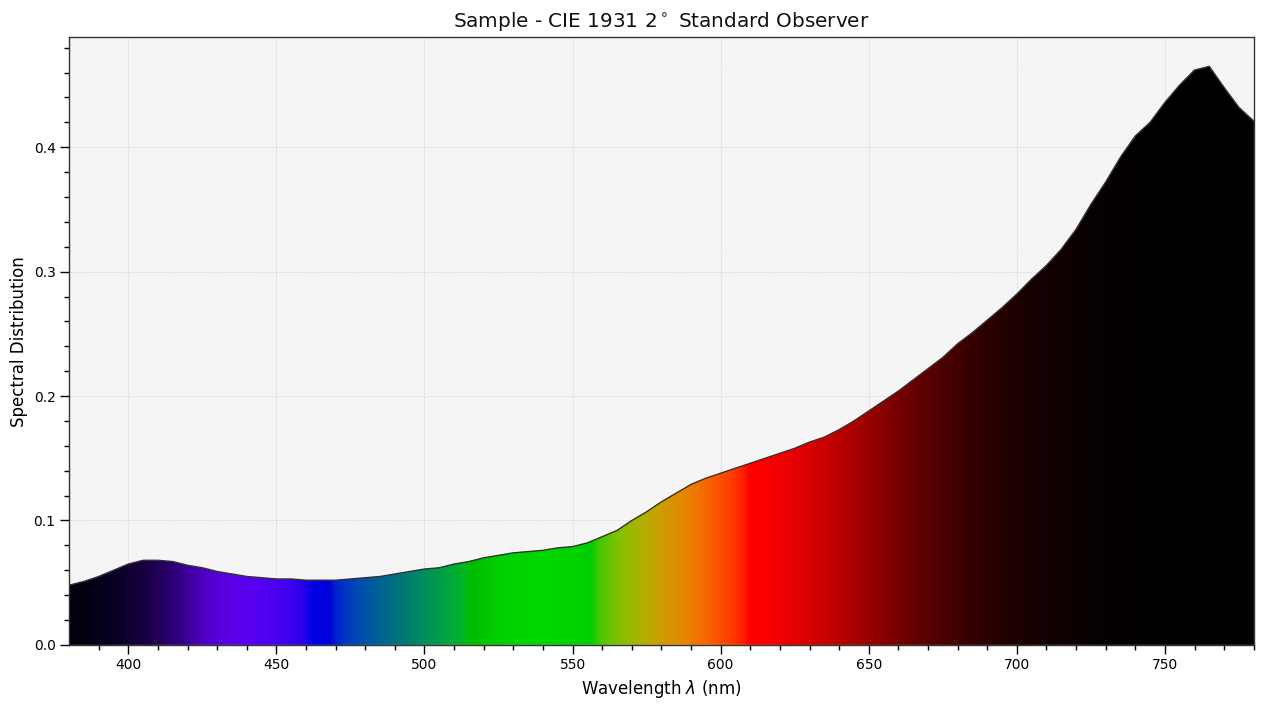With the sample spectral distribution defined, we can retrieve its shape:

In :
# Displaying the sample spectral distribution shape.
print(sd.shape)
(380.0, 780.0, 5.0)

The shape returned is an instance of colour.SpectralShape class:

In :
repr(sd.shape)
Out:
'SpectralShape(380.0, 780.0, 5.0)'

The colour.SpectralShape class is used throughout Colour to define spectral dimensions and is instantiated as follows:

In :
# Using *colour.SpectralShape* with iteration.
shape = colour.SpectralShape(start=0, end=10, interval=1)
for wavelength in shape:
print(wavelength)

# *colour.SpectralShape.range* method is providing the complete range of values.
shape = colour.SpectralShape(0, 10, 0.5)
shape.range()
0.0
1.0
2.0
3.0
4.0
5.0
6.0
7.0
8.0
9.0
10.0
Out:
array([  0. ,   0.5,   1. ,   1.5,   2. ,   2.5,   3. ,   3.5,   4. ,
4.5,   5. ,   5.5,   6. ,   6.5,   7. ,   7.5,   8. ,   8.5,
9. ,   9.5,  10. ])

Colour defines three convenient objects to create constant spectral distributions:

• colour.sd_constant
• colour.sd_zeros
• colour.sd_ones
In :
# Defining a constant spectral distribution.
constant_sd = colour.sd_constant(100)
print('"Constant Spectral Distribution"')
print(constant_sd.shape)
print(constant_sd)

# Defining a zeros filled spectral distribution.
print('\n"Zeros Filled Spectral Distribution"')
zeros_sd = colour.sd_zeros()
print(zeros_sd.shape)
print(zeros_sd)

# Defining a ones filled spectral distribution.
print('\n"Ones Filled Spectral Distribution"')
ones_sd = colour.sd_ones()
print(ones_sd.shape)
print(ones_sd)
"Constant Spectral Distribution"
(360.0, 780.0, 1.0)
100.0

"Zeros Filled Spectral Distribution"
(360.0, 780.0, 1.0)
0.0

"Ones Filled Spectral Distribution"
(360.0, 780.0, 1.0)
1.0

By default the shape used by colour.sd_constant, colour.sd_zeros and colour.sd_ones is the one defined by colour.DEFAULT_SPECTRAL_SHAPE attribute using the CIE 1931 2° Standard Observer shape.

In :
print(repr(colour.DEFAULT_SPECTRAL_SHAPE))
SpectralShape(360, 780, 1)

A custom shape can be passed to construct a constant spectral distribution with tailored dimensions:

In :
colour.sd_ones(colour.SpectralShape(400, 700, 5))
Out:
1.0

Often interpolation of the spectral distribution is needed, this is achieved with the colour.SpectralDistribution.interpolate method. Depending on the wavelengths uniformity, the default interpolation method will differ. Following CIE 167:2005 recommendation: The method developed by Sprague (1880) should be used for interpolating functions having a uniformly spaced independent variable and a Cubic Spline method for non-uniformly spaced independent variable. 

We can check the uniformity of the sample spectral distribution:

In :
# Checking the sample spectral distribution uniformity.
print(sd.is_uniform())
True

Since the sample spectral distribution is uniform the interpolation will be using the colour.SpragueInterpolator interpolator.

Note: Interpolation happens in place and may alter your original data, use the colour.SpectralDistribution.copy method to produce a copy of your spectral distribution before interpolation.

In :
# Copying the sample spectral distribution.
sd_copy = sd.copy()

# Interpolating the copied sample spectral distribution.
sd_copy.interpolate(colour.SpectralShape(400, 770, 1))
sd_copy
Out:
0.065809599999999996
In :
# Comparing the interpolated spectral distribution with the original one.
plot_multi_sds([sd, sd_copy], bounding_box=[730,780, 0.1, 0.5]);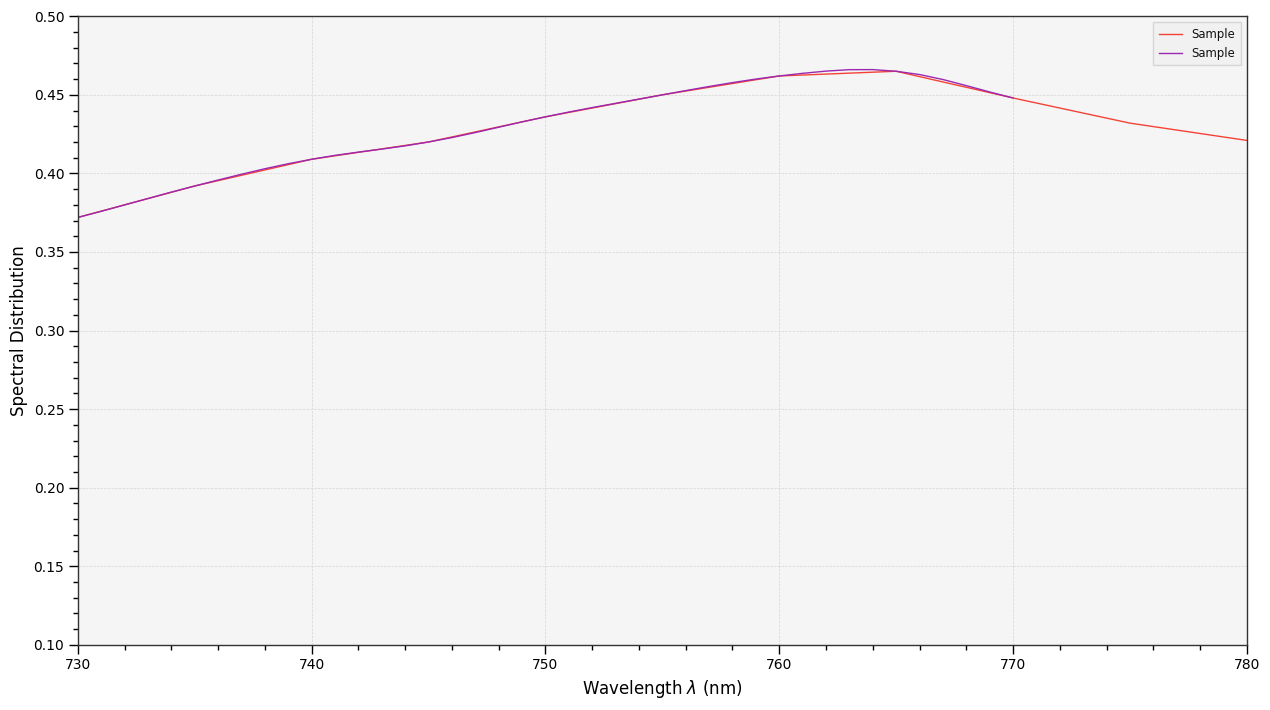Extrapolation although dangerous can be used to help aligning two spectral distributions together. CIE 015:2004 Colorimetry, 3rd Edition recommends that unmeasured values may be set equal to the nearest measured value of the appropriate quantity in truncation: 

In :
# Extrapolating the copied sample spectral distribution.
sd_copy.extrapolate(colour.SpectralShape(340, 830))
sd_copy, sd_copy
Out:
(0.064999999999999947, 0.44800000000000018)

The underlying interpolator can be swapped for any of the Colour interpolators.

In :
pprint([
export for export in colour.algebra.interpolation.__all__
if 'Interpolator' in export
])
['KernelInterpolator',
'NearestNeighbourInterpolator',
'LinearInterpolator',
'SpragueInterpolator',
'CubicSplineInterpolator',
'PchipInterpolator',
'NullInterpolator']
In :
# Changing interpolator while trimming the copied spectral distribution.
sd_copy.interpolate(
colour.SpectralShape(400, 700, 10), interpolator=colour.LinearInterpolator)
Out:
SpectralDistribution([[  4.00000000e+02,   6.50000000e-02],
[  4.10000000e+02,   6.80000000e-02],
[  4.20000000e+02,   6.40000000e-02],
[  4.30000000e+02,   5.90000000e-02],
[  4.40000000e+02,   5.50000000e-02],
[  4.50000000e+02,   5.30000000e-02],
[  4.60000000e+02,   5.20000000e-02],
[  4.70000000e+02,   5.20000000e-02],
[  4.80000000e+02,   5.40000000e-02],
[  4.90000000e+02,   5.70000000e-02],
[  5.00000000e+02,   6.10000000e-02],
[  5.10000000e+02,   6.50000000e-02],
[  5.20000000e+02,   7.00000000e-02],
[  5.30000000e+02,   7.40000000e-02],
[  5.40000000e+02,   7.60000000e-02],
[  5.50000000e+02,   7.90000000e-02],
[  5.60000000e+02,   8.70000000e-02],
[  5.70000000e+02,   1.00000000e-01],
[  5.80000000e+02,   1.15000000e-01],
[  5.90000000e+02,   1.29000000e-01],
[  6.00000000e+02,   1.38000000e-01],
[  6.10000000e+02,   1.46000000e-01],
[  6.20000000e+02,   1.54000000e-01],
[  6.30000000e+02,   1.63000000e-01],
[  6.40000000e+02,   1.73000000e-01],
[  6.50000000e+02,   1.88000000e-01],
[  6.60000000e+02,   2.04000000e-01],
[  6.70000000e+02,   2.22000000e-01],
[  6.80000000e+02,   2.42000000e-01],
[  6.90000000e+02,   2.61000000e-01],
[  7.00000000e+02,   2.82000000e-01]],
interpolator=SpragueInterpolator,
interpolator_args={},
extrapolator=Extrapolator,
extrapolator_args={'method': 'Constant', 'left': None, 'right': None})

The extrapolation behaviour can be changed for Linear method instead of the Constant default method or even use arbitrary constant left and right values:

In :
# Extrapolating the copied sample spectral distribution with *Linear* method.
sd_copy.extrapolate(
colour.SpectralShape(340, 830),
extrapolator_args={'method': 'Linear',
'right': 0})
sd_copy, sd_copy
Out:
(0.046999999999999341, 0.0)

Aligning a spectral distribution is a convenient way to first interpolate the current data within its original bounds then if needed extrapolates any missing values to match the requested shape:

In :
# Aligning the cloned sample spectral distribution.
# We first trim the spectral distribution as above.
sd_copy.interpolate(colour.SpectralShape(400, 700))
sd_copy.align(colour.SpectralShape(340, 830, 5))
sd_copy, sd_copy
Out:
(0.064999999999999974, 0.28199999999999986)

The colour.SpectralDistribution class also supports various arithmetic operations like addition, subtraction, multiplication, division or exponentiation with numeric and array_like variables or other colour.SpectralDistribution class instances:

In :
sd = colour.SpectralDistribution({
410: 0.25,
420: 0.50,
430: 0.75,
440: 1.0,
450: 0.75,
460: 0.50,
480: 0.25
})

print((sd.copy() + 1).values)
print((sd.copy() * 2).values)
print((sd * [0.35, 1.55, 0.75, 2.55, 0.95, 0.65, 0.15]).values)
print((sd * colour.sd_constant(2, sd.shape) * colour.sd_constant(3, sd.shape)).values)
[ 1.25  1.5   1.75  2.    1.75  1.5   1.25]
[ 0.5  1.   1.5  2.   1.5  1.   0.5]
[ 0.0875  0.775   0.5625  2.55    0.7125  0.325   0.0375]
[ 1.5  3.   4.5  6.   4.5  3.   nan  1.5]

The spectral distribution can be normalised with an arbitrary factor:

In :
print(sd.normalise().values)
print(sd.normalise(100).values)
[ 0.25  0.5   0.75  1.    0.75  0.5   0.25]
[  25.   50.   75.  100.   75.   50.   25.]

Colour Matching Functions¶

In the late 1920's, Wright (1928) and Guild (1931) independently conducted a series of colour matching experiments to quantify the colour ability of an average human observer which laid the foundation for the specification of the CIE XYZ colourspace. The results obtained were summarized by the Wright & Guild 1931 2° RGB CMFs $\bar{r}(\lambda)$,$\bar{g}(\lambda)$,$\bar{b}(\lambda)$ colour matching functions: they represent the amounts of three monochromatic primary colours $\textbf{R}$,$\textbf{G}$,$\textbf{B}$ needed to match the test colour at a single wavelength of light.

In :
# Plotting *Wright & Guild 1931 2 Degree RGB CMFs* colour matching functions.
plot_single_cmfs('Wright & Guild 1931 2 Degree RGB CMFs');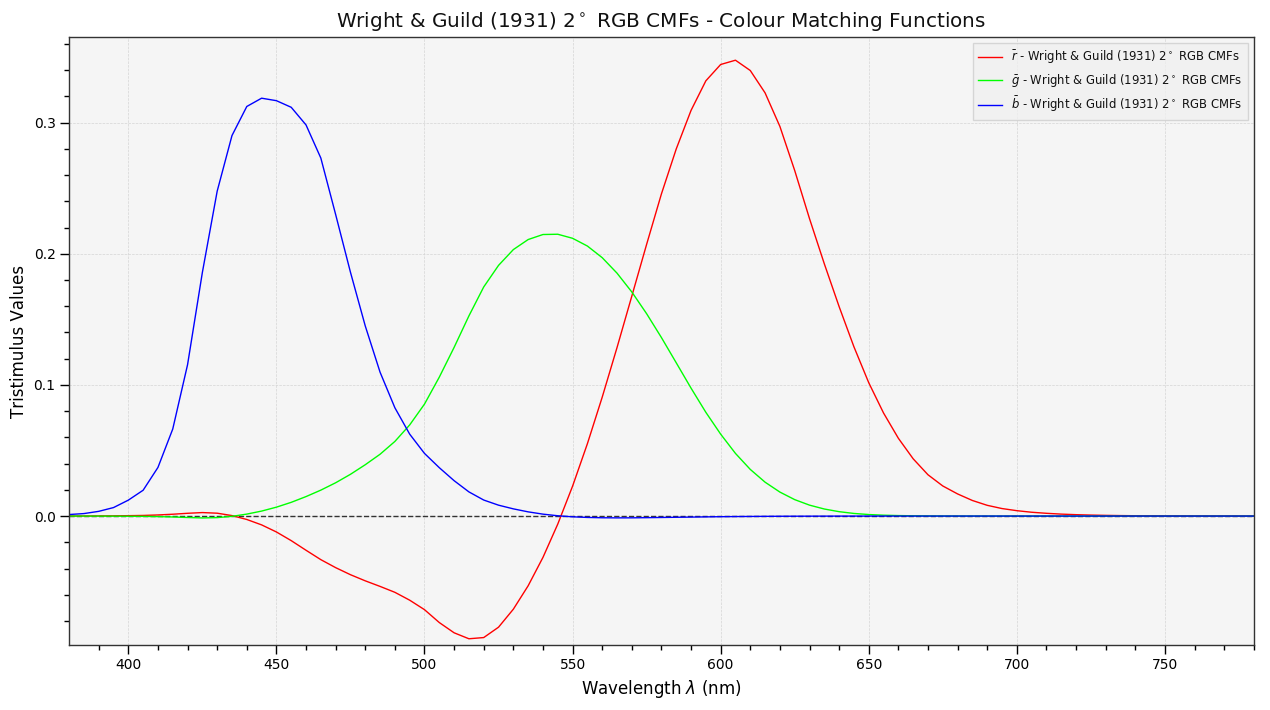With an RGB model of human vision based on Wright & Guild 1931 2° RGB CMFs $\bar{r}(\lambda)$,$\bar{g}(\lambda)$,$\bar{b}(\lambda)$ colour matching functions and for pragmatic reasons the CIE members developed a new colour space that would relate to the CIE RGB colourspace but for which all tristimulus values would be positive for real colours: CIE XYZ described with $\bar{x}(\lambda)$,$\bar{y}(\lambda)$,$\bar{z}(\lambda)$ colour matching functions.

In :
# Plotting *CIE XYZ 1931 2 Degree Standard Observer* colour matching functions.
plot_single_cmfs('CIE 1931 2 Degree Standard Observer');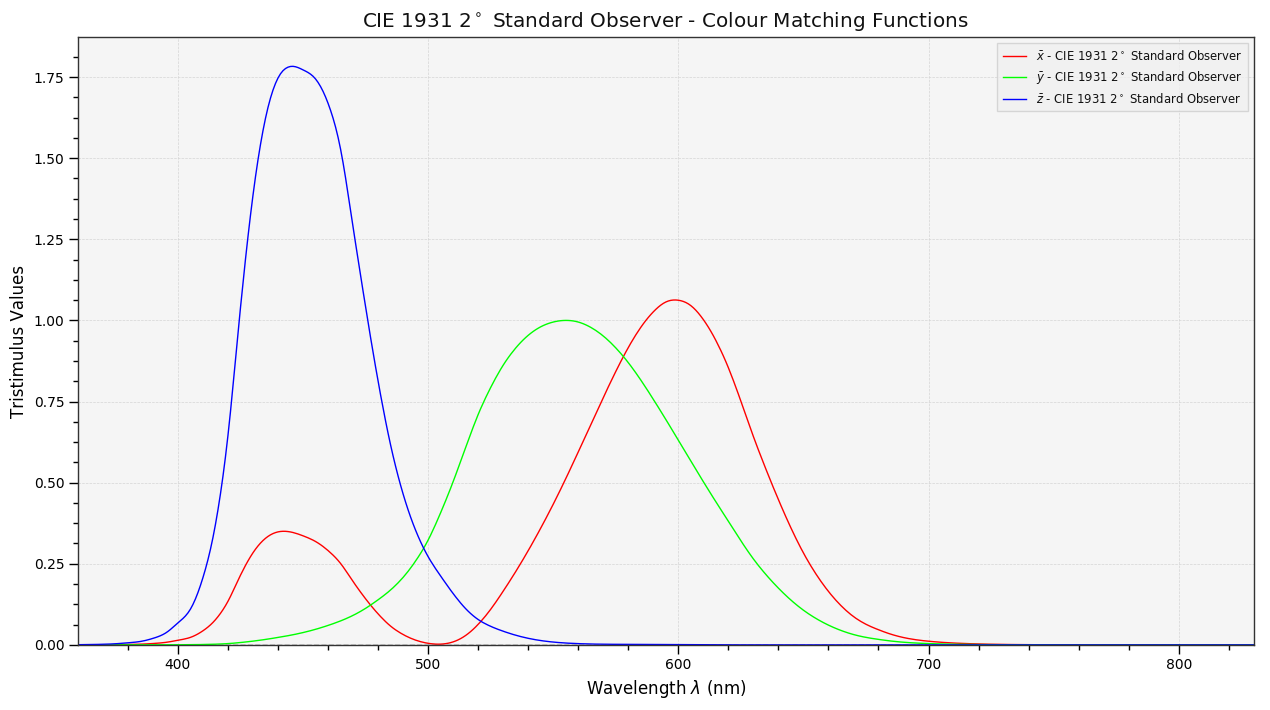In the 1960's it appeared that cones were present in a larger region of eye than the one initially covered by the experiments that lead to the CIE 1931 2° Standard Observer specification.

As a result, colour computations done with the CIE 1931 2° Standard Observer do not always correlate to the visual observation.

In 1964, the CIE defined an additional standard observer: the CIE 1964 10° Standard Observer derived from the work of Stiles and Burch (1959), and Speranskaya (1959). The CIE 1964 10° Standard Observer is believed to be a better representation of the human vision spectral response and recommended when dealing with a field of view of more than 4°.

For example and as per CIE recommendation, the CIE 1964 10° Standard Observer is commonly used with spectrophotometers for colour measurements whereas colorimeters generally use the CIE 1931 2° Standard Observer for quality control and other colour evaluation applications.

CIE XYZ Tristimulus Values¶

The CIE XYZ tristimulus values specify a colour stimulus in terms of the visual system. Their values for colour of a surface with spectral reflectance $\beta(\lambda)$ under an illuminant of spectral $S(\lambda)$ are calculated using the following equations: 

$$\begin{equation} X=k\int_{\lambda}\beta(\lambda)S(\lambda)\bar{x}(\lambda)d\lambda\\ Y=k\int_{\lambda}\beta(\lambda)S(\lambda)\bar{y}(\lambda)d\lambda\\ Z=k\int_{\lambda}\beta(\lambda)S(\lambda)\bar{z}(\lambda)d\lambda \end{equation}$$

where $$\begin{equation} k=\cfrac{100}{\int_{\lambda}S(\lambda)\bar{y}(\lambda)d\lambda} \end{equation}$$

However in virtually all practical computations of CIE XYZ tristimulus values, the integrals are replaced by summations:

$$\begin{equation} X=k\sum\limits_{\lambda=\lambda_a}^{\lambda_b}\beta(\lambda)S(\lambda)\bar{x}(\lambda)\Delta\lambda\\ Y=k\sum\limits_{\lambda=\lambda_a}^{\lambda_b}\beta(\lambda)S(\lambda)\bar{y}(\lambda)\Delta\lambda\\ Z=k\sum\limits_{\lambda=\lambda_a}^{\lambda_b}\beta(\lambda)S(\lambda)\bar{z}(\lambda)\Delta\lambda\\ \end{equation}$$

where $$\begin{equation} k=\cfrac{100}{\sum\limits_{\lambda=\lambda_a}^{\lambda_b}S(\lambda)\bar{y}(\lambda)\Delta\lambda} \end{equation}$$

Calculating the CIE XYZ tristimulus values of a colour stimulus is done using the colour.sd_to_XYZ definition which follows ASTM E2022–11 and ASTM E308–15 practises computation method:

In :
sd = colour.SpectralDistribution(sample_sd_data, name='Sample')
cmfs = colour.STANDARD_OBSERVERS_CMFS['CIE 1931 2 Degree Standard Observer']
illuminant = colour.ILLUMINANTS_SDS['A']

# Calculating the sample spectral distribution *CIE XYZ* tristimulus values.
colour.sd_to_XYZ(sd, cmfs, illuminant)
Out:
array([ 14.78676625,  10.97815867,   1.99023459])

Note: Output CIE XYZ colourspace matrix is in domain [0, 100].

CIE XYZ tristimulus values can be plotted into the CIE 1931 Chromaticity Diagram:

In :
import pylab

# Plotting the *CIE 1931 Chromaticity Diagram*.
# The argument *standalone=False* is passed so that the plot doesn't get displayed
# and can be used as a basis for other plots.
plot_chromaticity_diagram_CIE1931(standalone=False)

# Calculating the *xy* chromaticity coordinates.
# The output domain of *colour.spectral_to_XYZ* is [0, 100] and
# the input domain of *colour.XYZ_to_sRGB* is [0, 1].
# We need to take it in account and rescale the input *CIE XYZ* colourspace matrix.
x, y = colour.XYZ_to_xy(colour.sd_to_XYZ(sd, cmfs, illuminant) / 100)

# Plotting the *xy* chromaticity coordinates.
pylab.plot(x, y, 'o-', color='white')

# Annotating the plot.
pylab.annotate(sd.name,
xy=(x, y),
xytext=(-50, 30),
textcoords='offset points',

# Displaying the plot.
render(standalone=True);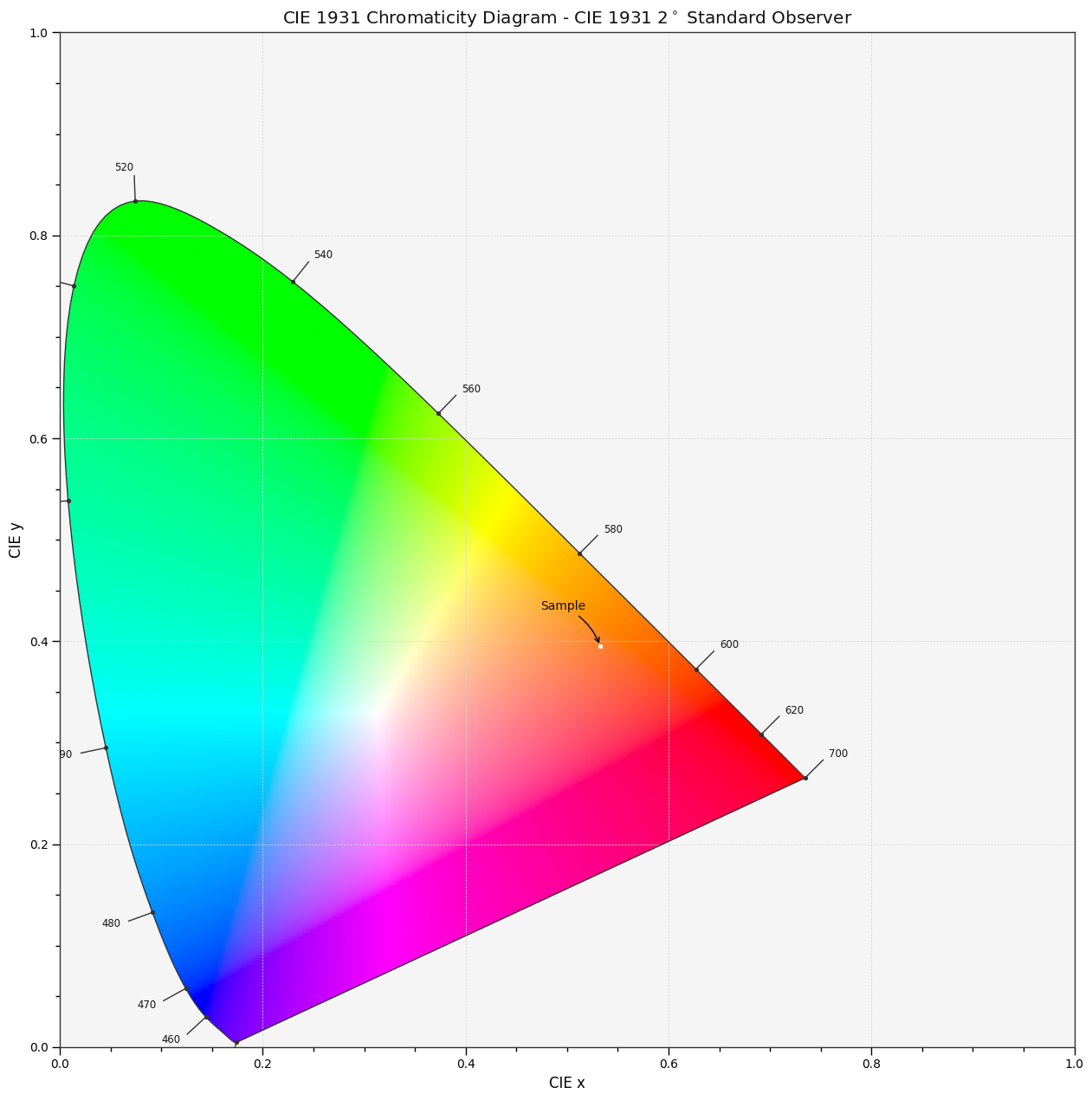Retrieving the CIE XYZ tristimulus values of any wavelength from colour matching functions is done using the colour.wavelength_to_XYZ definition, if the value requested is not available, the colour matching functions will be interpolated following CIE 167:2005 recommendation:

In :
colour.wavelength_to_XYZ(546.1, colour.STANDARD_OBSERVERS_CMFS['CIE 1931 2 Degree Standard Observer'])
Out:
array([ 0.3755316 ,  0.98444552,  0.01220285])

Bibliography¶

1. ^ CIE. (n.d.). 17-198 colour (perceived). Retrieved June 26, 2014, from http://eilv.cie.co.at/term/198
2. ^ CIE. (n.d.). 17-659 light. Retrieved June 26, 2014, from http://eilv.cie.co.at/term/659
3. ^ CIE. (n.d.). 17-1238 spectrum. Retrieved June 27, 2014, from http://eilv.cie.co.at/term/1238
4. ^ CIE TC 1-38. (2005). 9. INTERPOLATION. In CIE 167:2005 Recommended Practice for Tabulating Spectral Data for Use in Colour Computations (pp. 14–19). ISBN:978-3-901-90641-1
5. ^ CIE TC 1-48. (2004). CIE 015:2004 Colorimetry, 3rd Edition. CIE 015:2004 Colorimetry, 3rd Edition (pp. 1–82). ISBN:978-3-901-90633-6
6. ^ Wyszecki, G., & Stiles, W. S. (2000). Integration Replace by Summation. In Color Science: Concepts and Methods, Quantitative Data and Formulae (pp. 158–163). Wiley. ISBN:978-0471399186